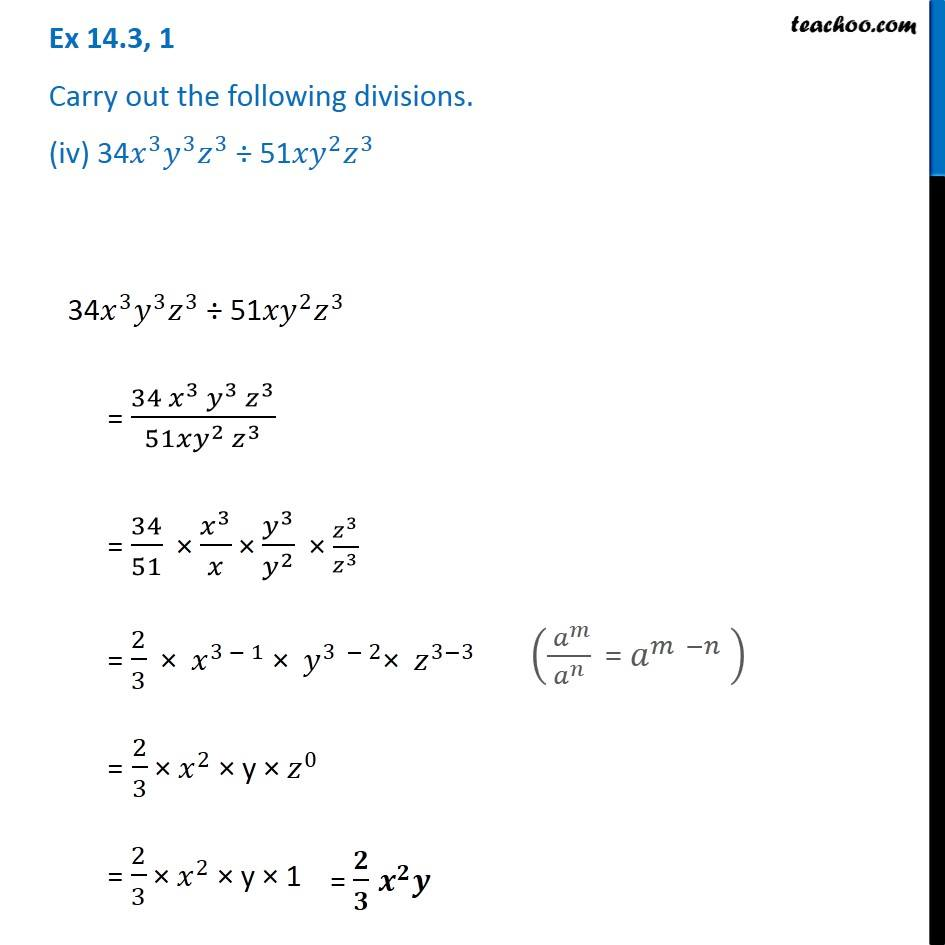1. Chapter 14 Class 8 Factorisation
2. Serial order wise
3. Ex 14.3

Transcript

Ex 14.3, 1 Carry out the following divisions. (iv) 34𝑥^3 𝑦^3 𝑧^3 ÷ 51〖𝑥𝑦〗^2 𝑧^3 34𝑥^3 𝑦^3 𝑧^3 ÷ 51〖𝑥𝑦〗^2 𝑧^3 = (34 𝑥^3 𝑦^3 𝑧^3)/(51𝑥𝑦^2 𝑧^3 ) = 34/51 × 𝑥^3/𝑥 × 𝑦^3/𝑦^2 × 𝑧^3/𝑧^3 = 2/3 × 𝑥^(3 − 1) × 𝑦^(3 − 2)× 𝑧^(3−3) = 2/3 × 𝑥^2 × y × 𝑧^0 = 2/3 × 𝑥^2 × y × 1 (( 𝑎^𝑚)/𝑎^𝑛 " = " 𝑎^(𝑚 −𝑛) " " ) = 𝟐/𝟑 𝒙^𝟐 𝒚

Ex 14.3